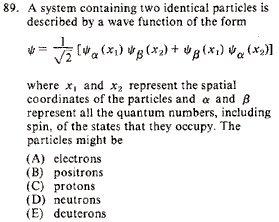GR 8677927796770177 | # Login | Register

GR8677 #89
Problem
 GREPhysics.NET Official Solution Alternate SolutionsQuantum Mechanics}Identical Particles

According to Griffiths, the proof to this rule comes from QFT,

where it's for bosons and for fermions.

The given identical particle wave function contains a plus sign, so the particles must be bosons. Bosons have integer spin (while fermions have half-integer spin). Electrons, protons, and neutrons are all fermions. A positron is just a positive electron, so that is presumably, also, a fermion. Thus, the remaining choice would be deuteron---which is a boson.Alternate Solutions
 casseverhart132019-08-22 07:12:09 Your way of putting problem together is admirable. paint contractor OrlandoReply to this commentdjh1012014-08-28 17:41:29 Let the two particles be in the same state. Note that the wave function is not 0. Particles must be bosons. A-D are fermions. Therefore, the answer is E.Reply to this commentcasseverhart13
2019-08-22 07:12:09
Your way of putting problem together is admirable. paint contractor Orlandodjh101
2014-08-28 17:41:29
Let the two particles be in the same state. Note that the wave function is not 0. Particles must be bosons. A-D are fermions. Therefore, the answer is E.justin_l
2012-11-08 15:00:07
If you didn't know what were fermionic and baryonic, you could think that electrons and positrons must be the 'same' category, so they can't be the answer. Protons and neutrons are also the 'same' category. The only one left is E.lemaitre
2011-04-03 13:45:12
just another clarification...

protons and neutrons are baryons (contain 3 quarks) and are thus technically 'fermionic hadrons'

compare this with pions or kaons that are mesons (2 quarks) and are thus 'bosonic hadrons'

thus the only answer left is choice (E)Almno10
2010-11-12 19:08:17
The minus sign for fermions guarantees two cannot occupy the same state; for then, the wave function would be the difference of identical functions, and would be zero.

The +, - thing is because there are two ways to write a normalizable wave function for two particles in which the particles are indistinguishable. This fact PREDICTS the existence of fermions and bosons, not the other way around.doubledecker
2010-11-09 11:23:50
If you recognize that it's going to come down to the distinction between bosons and fermions, then you've just got to play a little "one of these things is not like the other."terry
2008-11-01 01:27:48
protons and neutrons are NOT fermions. They are hadrons, they do not have 1/2 interger spin.
 terry2008-11-01 14:35:52 actually i am wrong. protons are fermions. i was thinking of photons
 carle2572010-04-09 00:18:19 Photons are also bosons with spin 1.tin2019
2007-09-09 01:47:00
I would just like to stress that the reason why + refers to bosons and - to fermions is that the wave function of a system of particles has to be symetric for bosons and antisymetric for fermions upon interchange of two particles. That means that we can write the solution as the product of single particle states which are the solution of schrodinger equation, and since the sum of the solution is also a solution we can form a sum such that the resulting wave function is symetric or antisymeric accordingly. See W. Greiner Introduction to Quantum Mechanics ). This being said one can deduce that if fermion system of particles having an antisymetric wave function were to contain two particles in the same staste psi(x1,x2,...,xk,...,xk,..xn), upon interchange of the two identical particles in the same state we get psi(x1,x2,...,xk,...,xk,..xn)=-psi(x1,x2,...,xk,...,xk,..xn) which implies that the wave funtion vanishes, i.e. no two fermions can exist in the same quantum state. This is just the generalization of Pauli exclusion principle.LaTeX syntax supported through dollar sign wrappers $, ex.,$\alpha^2_0$produces . type this... to get...$\int_0^\infty\partial\Rightarrow\ddot{x},\dot{x}\sqrt{z}\langle my \rangle\left( abacadabra \right)_{me}\vec{E}\frac{a}{b}\$